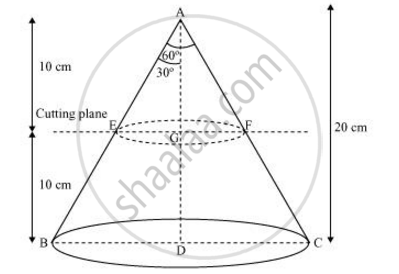Share

# A metallic right circular cone 20 cm high and whose vertical angle is 60° is cut into two parts at the middle of its height by a plane parallel to its base. - CBSE Class 10 - Mathematics

#### Question

A metallic right circular cone 20 cm high and whose vertical angle is 60° is cut into two parts at the middle of its height by a plane parallel to its base. If the frustum so obtained is drawn into a wire of diameter 1/16 cm, find the length of the wire [use π=22/7]

#### SolutionIn ΔAEG,

EG/AG =  tan 30º

EG = 10/sqrt3 cm = (10sqrt3)/3

In ΔABD,

BD = 20/sqrt3 =  (20sqrt3)/3cm

Radius (r1) of upper end of frustum = (10sqrt3)/3 cm

Radius (r2) of lower end of container  = (20sqrt3)/3cm

Height (h) of container = 10 cm

Volume of frustum = 1/3pih(r_1^2+r_2^2+r_1r_2)

=1/3xxpixx10[((10sqrt3)/3)^2 + ((20sqrt3)/3)^2 + ((10sqrt3)(10sqrt3))/(3xx3)]

= 10/3pi[100/3+400/3+200/3]

=10/3xx22/7xx700/3=22000/9 cm^3

Radius (r) of wire =  = 1/16xx1/2 = 1/32cm

Let the length of wire be l.

Volume of wire = Area of cross-section × Length

= (πr2) (l)

=pi(1/32)^2 xx l

Volume of frustum = Volume of wire

22000/9 = 22/7xx(1/32)^2xxl

7000/9 xx1024 = l

l = 796444.44 cm

= 7964.44 meters

Is there an error in this question or solution?

#### APPEARS IN

NCERT Solution for Mathematics Textbook for Class 10 (2019 to Current)
Chapter 13: Surface Areas and Volumes
Ex. 13.40 | Q: 5 | Page no. 257

#### Video TutorialsVIEW ALL 

Solution A metallic right circular cone 20 cm high and whose vertical angle is 60° is cut into two parts at the middle of its height by a plane parallel to its base. Concept: Frustum of a Cone.
S